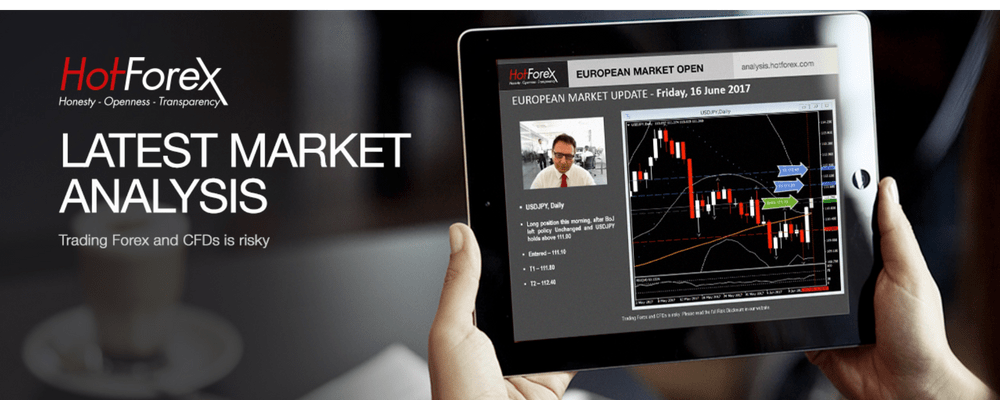# Assessing the long-term trend: Interest Rate DifferentialsAlthough sometimes ignored, having an understanding of the long-term trend can be important in crafting a successful investment tactic. An estimate of the trend, using either technical or fundamental analysis, can be obtained in many ways. Drawing from the fundamental pool of instruments, the remainder of this article provides an overview of how interest rate differentials can provide a relatively easy way of assessing long-term trends in currency pairs.

Defining the International Fisher Effect (IFE)

Back in 1930, economist Irving Fisher, whose work has returned to the limelight in the aftermath of the global financial crisis, was the first to put down the foundations of a relationship between interest rates and inflation. More specifically, Fisher commented that a country’s inflation rate will have an effect on a country’s interest rates. In particular, Fisher suggested that the real interest rate, i.e. interest rate excluding inflation, is, by definition, not affected by the inflation rate, and was assumed to be more-or-less the same across countries. However, the market interest rate, defined as the real interest rate plus inflation, should be indicative of the level market participants expect inflation to be in that particular country. To put Fisher’s proposal in perspective, suppose that the market interest rate in Japan is at 4% and the market interest rate in the US is at 3%. Given a stable real interest rate, inflation in Japan should then be 1% higher than inflation in the US. In honour of its creator, this has been named the International Fisher Effect (IFE).

The most important point regarding the Fisher effect is that it can be used for future interest rates and hence gauge anticipated inflation. For example, one can employ the 3-month interest rate in Japan and the US in order to gauge what the market expects the inflation to be in the coming three months. Given the importance of inflation in the currency market, the difference between two countries’ future interest rates can provide guidance as regards to the potential path of currency pair.

What works and what doesn’t

Before using the IFE in practice, one should remember an important point: in the years since the 1930s, interest rate policy has been mainly determined by central banks which set interest rates based on the phase of the business cycle. In essence, central banks tend to increase interest rates when the economy is growing, in order not to overheat, and decrease interest rates when the economy is losing speed. Fisher’s argument would only hold globally if the whole world was facing the same economic cycle. In other words, if the Japanese economy is stagnating and the US economy is booming then BoJ interest rates would be lower than Fed interest rates as a result of the different phase in the cycle. This would then cloud the original definition of the Fisher effect as the result would not reflect just differences in the inflation rate. This would explain why economists have found mixed results in regards to the effectiveness of the Fisher effect.

Further to this, the other problem with the IFE is that its assumption of similar real rates was found not to hold in practice. In real life, higher interest rates tend to have an appreciative effect on the value of a country’s currency, as they usually attract more foreign investment given that returns are expected to be higher than in countries with lower interest rates. This increases the demand for the country’s currency and hence makes the interest rate appreciate. In a similar manner, lower interest rates tend to discourage foreign investment, lower demand for the currency and depreciate the exchange rate. Hence, what would really hold in practice would be the inverse of the IFE, which, for simplicity, we will denote as IIFE (Inverse International Fisher Effect).

An illustrative example

To view how the IIFE works in practice, the chart shows the Libor interest rate differential between the US and the UK, after excluding the policy interest rate.* The Chart is also adjusted so that the present move in the exchange rate coincides with the interest rate differential six months ago. Data arrows show the trends for the GBPUSD (purple) and the 6-month differential (green). Remember that the interest rate differential is positive when the US rate is higher than the respective UK rate, which should indicate a fall in the GBPUSD rate.

To illustrate how the exchange rate moves in the opposite direction of the interest rate differential, note that as the blue line moves from point 1 to point 2, the exchange rate declines from point A to point B. In a similar manner, the decline in the differential from point 2 to point 3 is followed by an increase from point B to point C. From point 3 onward, the positive interest rate differential again leads to a decrease in the GBPUSD rate.

Note that interest rate differentials are only indicative of the long-term trend and, despite what the original IFE may suggest, it is very difficult to use them for shorter-term forecasts. One should remember that forecasting short-term movements with longer-term indicators does not work well in practice. While some ups and downs of the differential can be associated with opposite movements of the exchange rate, and the overall correlation stands at -0.70, which is a quite large number (and for the statistically inclined, statistically significant at the 1% level in a regression estimate), importance should be placed on the longer-term trends, given that these are less prone to noise.

Investors and traders should remember that longer-term trends shouldn’t be overlooked. To this end, the IIFE provides a relatively easy way to obtain an indication of the long-term path of a currency pair.

*To perform this, data for the 6-month Libor on the Dollar and Sterling were used, deducting the overnight Libor rate. All data were obtained from US FRED.